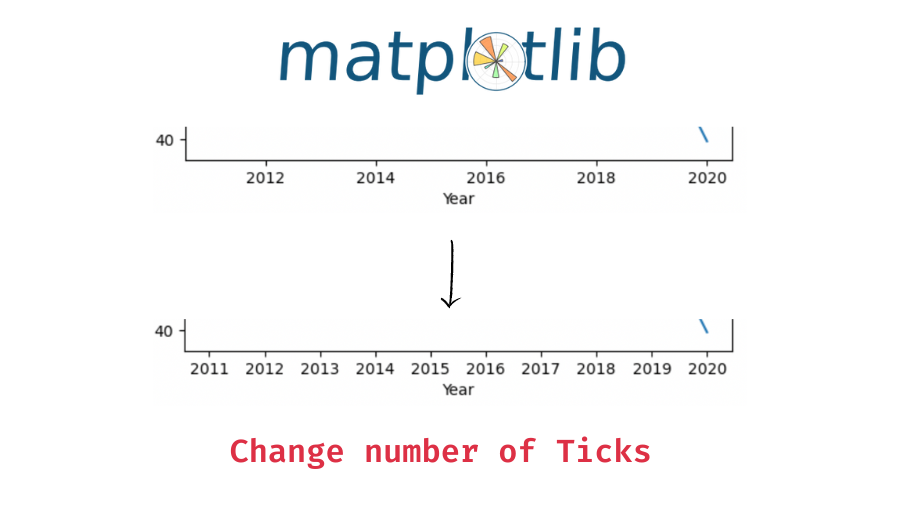# Matplotlib – Change the Number of Ticks in a Plot

In this tutorial, we will look at how to change the tick frequency in a matplotlib plot with the help of some examples.

You can change the number of ticks in matplotlib in the following two ways –

1. Using the `maplotlib.pyplot` object’s `xticks()` and the `yticks()` functions to modify the ticks on the x-axis and the y-axis respectively.
2. You can also use the `maplolib.pyplot` object’s `locator_params()` function to specify the number of ticks on a specific axis.

Let’s now look at both methods with some examples.

First, we will create a plot that we will use throughout this tutorial.

```import matplotlib.pyplot as plt

# x values - years
x = [2011, 2012, 2013, 2014, 2015, 2016, 2017, 2018, 2019, 2020]
# y values - curde oil price per barrel in USD
y = [94.88, 94.05, 97.98, 93.17, 48.66, 43.29, 50.80, 65.23, 56.99, 39.68]

# plot x and y on a line plot
plt.plot(x, y)

plt.xlabel('Year')
plt.ylabel('Crude oil price USD/barrel')```

Output:

## Example 1 – Change the number of ticks on the x-axis

The `matplotlib.pyplot.xticks()` function takes `ticks` and `labels` as parameters. You can pass the tick locations as an array to the `ticks` parameter and the corresponding tick labels to the `labels` parameter. In the above plot, we see that there are only 5 tick values, let’s now get a tick for each value in the `x` list.

```# plot x and y on a line plot
plt.plot(x, y)

plt.xlabel('Year')
plt.ylabel('Crude oil price USD/barrel')

# specify the ticks and its frequency
plt.xticks(x)

# draw the plot
plt.draw()```

Output:

There are now 10 ticks on the x-axis.

📚 Data Science Programs By Skill Level

Introductory

Intermediate ⭐⭐⭐

🔎 Find Data Science Programs 👨‍💻 111,889 already enrolled

Disclaimer: Data Science Parichay is reader supported. When you purchase a course through a link on this site, we may earn a small commission at no additional cost to you. Earned commissions help support this website and its team of writers.

Alternatively, we can use the `maplotlib.pyplot.locator_params()` function and only specify the number of ticks we want (instead of specifying the actual tick locations like above).

The `matplotlib.pylot.locator_params()` function takes `axis` and `nbins` as parameters. Specify the axis to which you want to apply these settings with the `axis` parameter and the number of ticks you want to the `nbins` parameter.

```# plot x and y on a line plot
plt.plot(x, y)

plt.xlabel('Year')
plt.ylabel('Crude oil price USD/barrel')

# specify the number of ticks on the x-axis
plt.locator_params(axis='x', nbins=10)

# draw the plot
plt.draw()```

Output:

We get the same result as above.

## Example 2 – Change the number of ticks on the y-axis

You can similarly change the number of ticks on the y-axis.

Use the `mapltolib.pyplot.yticks()` function to give custom ticks and tick labels to the plot. Let’s change the number of ticks on the y-axis to 3 in the original scatter plot.

```import numpy as np

# plot x and y on a line plot
plt.plot(x, y)

plt.xlabel('Year')
plt.ylabel('Crude oil price USD/barrel')

# specify use 3 equally spaced values between min and max values as yticks
plt.yticks(np.linspace(min(y), max(y), 3))

# draw the plot
plt.draw()```

Output:

The y-axis now has only 3 ticks.

Alternatively, use the `maplotlib.pyplot.locator_params()` functions and specify the number of ticks for the y-axis.

```# plot x and y on a line plot
plt.plot(x, y)

plt.xlabel('Year')
plt.ylabel('Crude oil price USD/barrel')

# specify the number of ticks on the y-axis
plt.locator_params(axis='y', nbins=3)

# draw the plot
plt.draw()```

Output:

## Example 3 – Changing the number of ticks on subplots

You can similarly change the number of ticks (on the x-axis and/or the y-axis) on subplots with the corresponding matplotlib axes object functions.

1. Use the axes object’s `set_xticks()` function to change the ticks on the x-axis and the `set_yticks()` function to change the ticks on the y-axis.
2. Alternatively, you can use the axes object’s `locator_params()` function.

Let’s look at an example –

```fig, (ax1, ax2) = plt.subplots(1, 2, figsize=(10, 5))

# plot the first subplot
ax1.plot(x, y)
ax1.set_xlabel('Year')
ax1.set_ylabel('Crude oil price USD/barrel')

# plot the second subplot
ax2.plot(x, y)
ax2.set_xlabel('Year')
ax2.set_ylabel('Crude oil price USD/barrel')```

Output:

Here, we created two subplots. Both the subplots have the same data but that is not important. Let’s see how to modify the number of ticks in the above subplots.

```fig, (ax1, ax2) = plt.subplots(1, 2, figsize=(10, 5))

# plot the first subplot
ax1.plot(x, y)
ax1.set_xlabel('Year')
ax1.set_ylabel('Crude oil price USD/barrel')
# change number of ticks to 10 on the x-axis
ax1.set_xticks(x)

# plot the second subplot
ax2.plot(x, y)
ax2.set_xlabel('Year')
ax2.set_ylabel('Crude oil price USD/barrel')
# change number of ticks to 10 on the x-axis
ax2.locator_params(axis='x', nbins=10)```

Output:

To change the number of ticks on the axis we used –

1. For the first subplot, the axes object’s `set_xticks()` function.
2. For the second subplot, the axes object’s `locator_params()` function.

You might also be interested in –

•